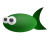## Symmetric transformations for floating point alpha

babl clamps the alpha used when going from separate alpha to associated alpha or from associated alpha to separate alpha to BABL_ALPHA_FLOOR. This replaces asymptotic behavior and direct precision loss of color precision when multiplying or dividing by alphas near 0.0 with a consistent symmetric transformation.

Original intent of data as well as non-asymptotic precision loss is thus maintained when the processing chain might temporarily use the other alpha representation.

```    #define BABL_ALPHA_FLOOR    (1.0/65536.0)
#define BABL_ALPHA_FLOOR_F  (1.0f/65536.0f)
```

The deviation from not clamping near 0.0 is within the quantization margin of 16bit integer alpha, thus no adaptations for any SIMD or and similar 8bit and 16bit extensions of pixel format conversions are needed.

This is the clamping function in use:

```static inline float
babl_epsilon_for_zero_float (float value)
{
if (value <= BABL_ALPHA_FLOOR_F &&
value >= -BABL_ALPHA_FLOOR_F)
return BABL_ALPHA_FLOOR_F;
else
return value;
}
```

And an example use of this clamping function that is consistent with babls behavior:

```static inline void
associated_to_separate_rgba (const float *associated_rgba,
float *separate_rgba)
{
float alpha = associated_rgba;
float reciprocal_alpha = 1.0f / babl_epsilon_for_zero_float (alpha);

separate_rgba = associated_rgba * reciprocal_alpha;
separate_rgba = associated_rgba * reciprocal_alpha;
separate_rgba = associated_rgba * reciprocal_alpha;
separate_rgba = alpha;
}

static inline void
separate_to_associated_rgba (const float *separate_rgba,
float *associated_rgba)
{
float alpha = associated_rgba;
float limited_alpha = babl_epsilon_for_zero_float (alpha);

associated_rgba = separate_rgba * limited_alpha;
associated_rgba = separate_rgba * limited_alpha;
associated_rgba = separate_rgba * limited_alpha;
associated_rgba = alpha;
}
```

For more detils see the commit message of the most recent refinement as well as blog post with further background.